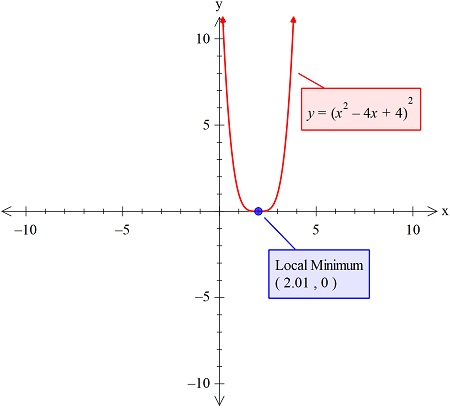# Find the relative maxima and minima, if any, for the following function: f(x)=(x^2-4x+4)^2

## Question:

Find the relative maxima and minima, if any, for the following function:

{eq}f(x)=(x^2-4x+4)^2 {/eq}

## Finding Minima & Maxima:

The maxima or the function is not possible is the function's curve is stretching till infinity, however, the minima of the curve can be possible if there is any turning point of the graph of the function.

Here we have the complex function, so we can use the graphical approach and plot the function's curve using the graphing utility.

So the curve is:So here we have no maxima but just the minimum at point (2.01,1)